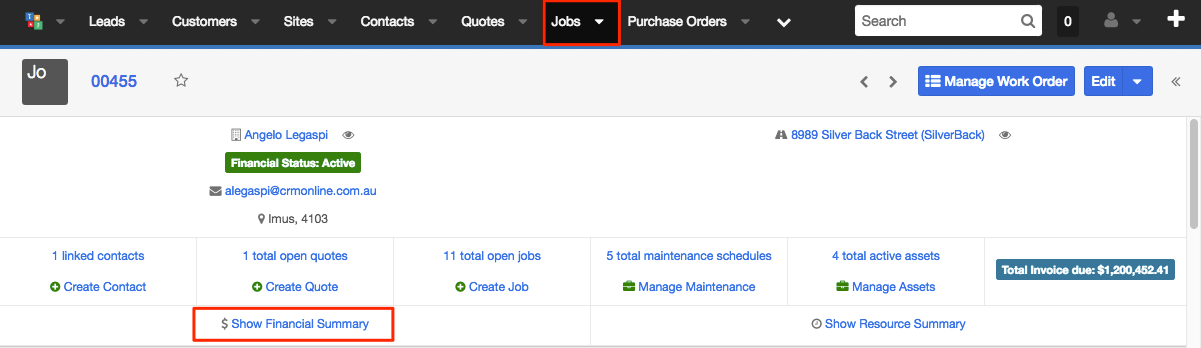## USING THE FINANCIAL SUMMARY OF A JOB

Open a job record. In the single view simply click the "Show Financial Summary" link:It will display the following computed values:

• Amount - This refers to the job amount
• Total Invoiced - This is  the total amount that is invoiced against the job excluding tax
• Percent Invoiced Including Variance - Computed as
(Total Invoiced / Total Contract Value) * 100
• Total Labour is the Total of the time entries against the job. The time entry is computed as (Hours* Hourly rate)
• Percent Invoiced Including Variations- This is (Total Invoiced / Total Contract Value) * 100
• Total Labour - The total of the time entries against the job ( each time entry rate formula is hours * Hourly Rate)
• Gross Profit- This is Total Invoiced -Total Job Cost
• Percent Invoiced Excluding Variations -This is computed as follow:
• Simple and Project Job-100 - (100 *(Remaining Amount / Variation Amount))
• Recurring Job-100 - (100 * (Remaining Amount / Invoice Amount))
• Total Expenses- This is the Total Cost of Materials Used against the job + Total POs
• Total Job Cost- This is the Total Labour + Total Expenses
• Gross Margin(%)- This is  the ((Total Invoice Value (Ex GST) - Total Job Cost) / Total Invoice Value (Ex GST)) * 100## Get In Touch

Are you looking to streamline your field services business, or would just like to chat?

Fieldmagic for Android - Requires Android
Job Management Software
5.0 ( 1 ratings )
Price: \$29.00
Fieldmagic for iOS - Requires iOS
Field Service Software
5.0 ( 1 ratings )
Price: \$29.00